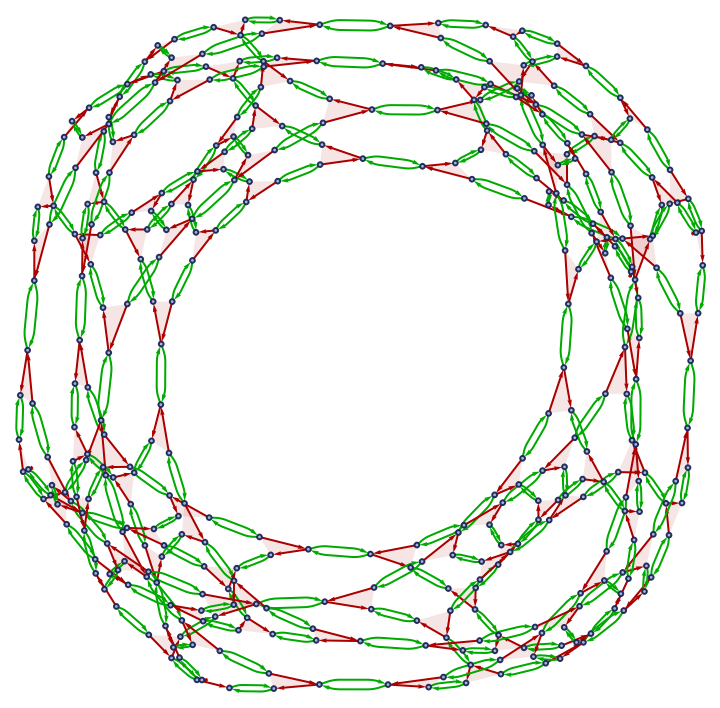Function Repository Resource:

WolframModelPlot

Generate a visual display of a hypergraph

Contributed by: Wolfram Research
 ResourceFunction["WolframModelPlot"][g] generates a plot of the hypergraph g. ResourceFunction["WolframModelPlot"][{g1,g2,…}] generates a list of plots of the hypergraphs gi. ResourceFunction["WolframModelPlot"][data,"type"] generates plots assuming hypergraphs of the specified type.

Details and Options

ResourceFunction["WolframModelPlot"] attempts to place vertices to give a well-laid-out version of the hypergraph.
In ResourceFunction["WolframModelPlot"][g], g should be a list of hyperedges: {{n11,n12,},{n21,},}.
Unary edges are supported and displayed as circles around the vertices.
In ResourceFunction["WolframModelPlot"][data,"type"], possible types of hypergraphs to assume include:
 "Ordered" every hyperedge is interpreted as a sequence of directed edges of adjacent vertices "Cyclic" the same as "Ordered", with an addition of a directed edge from the last vertex to the first
WolframModelPlot has the same options as Graphics, with following additions:
 "ArrowheadLength" the length of arrowheads "EdgePolygonStyle" style for hyperedge polygons "HyperedgeRendering" "Polygons" how to render hyperedges in the hypergraph "MaxImageSize" upper limit for the image size style for edges {} vertices and hyperedges to highlight highlight color overall style {} coordinates specified for particular vertices labels for vertices 0.06 the size of vertices style for vertices
Possible settings for "HyperedgeRendering" include:
 "Subgraphs" render hyperedges as ordinary graphs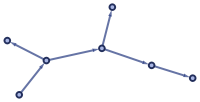"Polygons" render hyperedges as polygons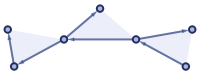Install the SetReplace paclet to get better performance and bleeding-edge functionality.
See the SetReplace README for a more detailed documentation.

Examples

Basic Examples (3)

Plot a hypergraph:

 In:=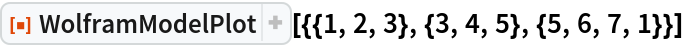Out=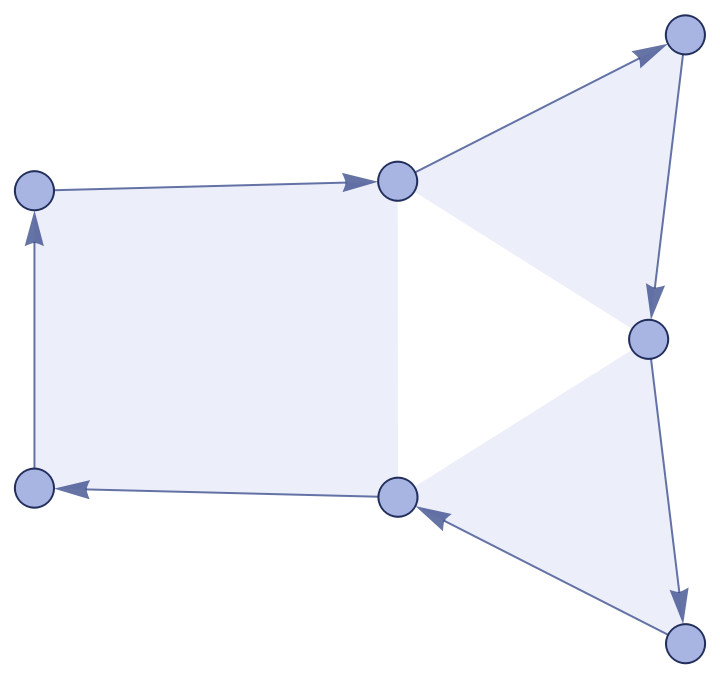Plot multiple hypergraphs:

 In:=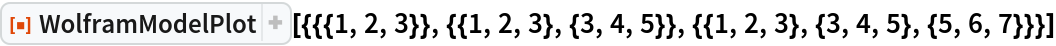Out=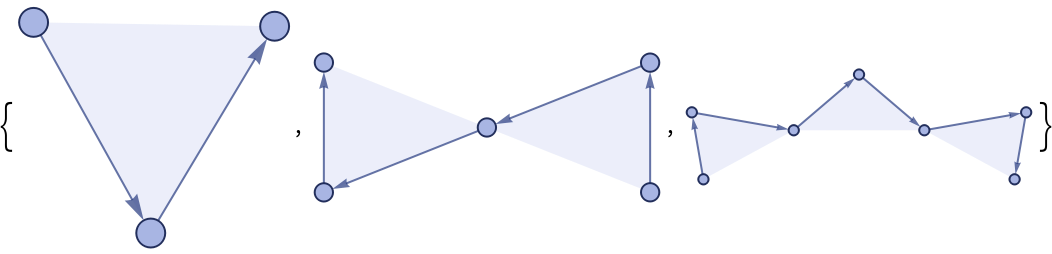Plot a cyclic hypergraph:

 In:=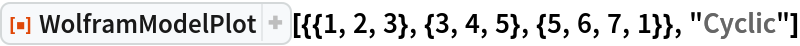Out=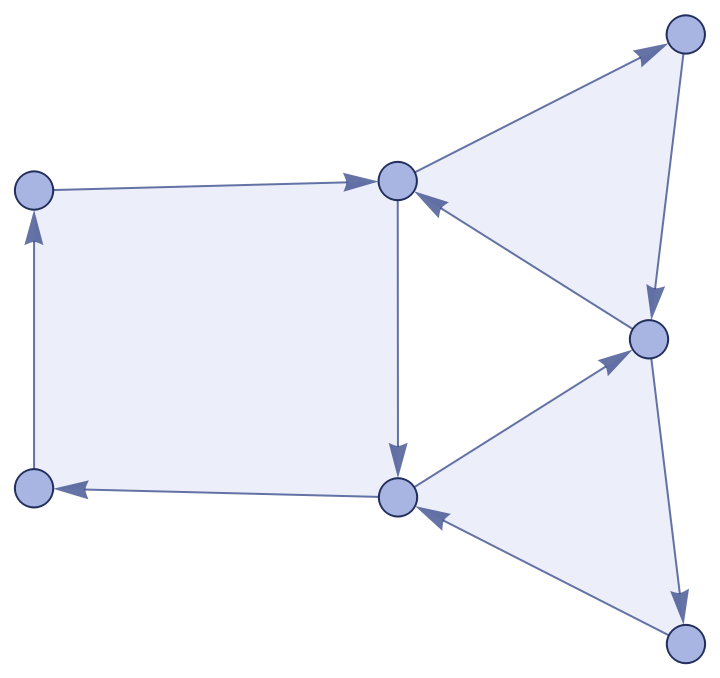Scope (4)

Unary edges are shown as circles around the vertices:

 In:=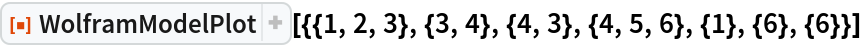Out=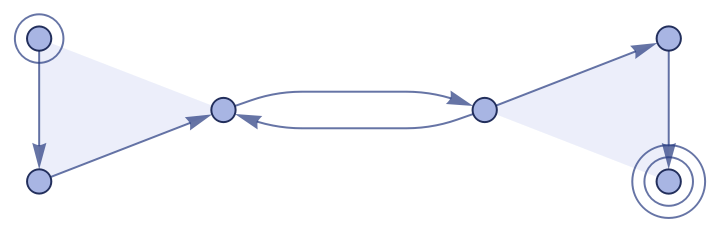Self-loops are shown as convex polygons around the appropriate number of circular arrows:

 In:=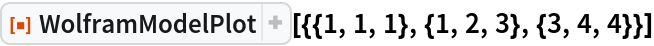Out=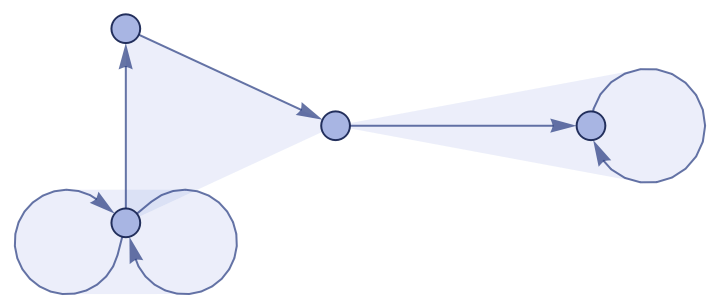Note the difference between a hyper-self-loop and two binary edges pointing in opposite directions:

 In:=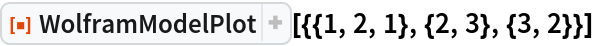Out=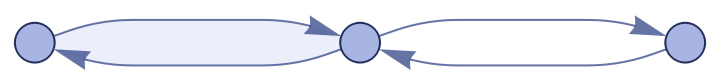Multiedges are shown as multiple arrows and polygons:

 In:=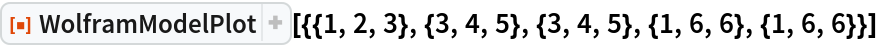Out=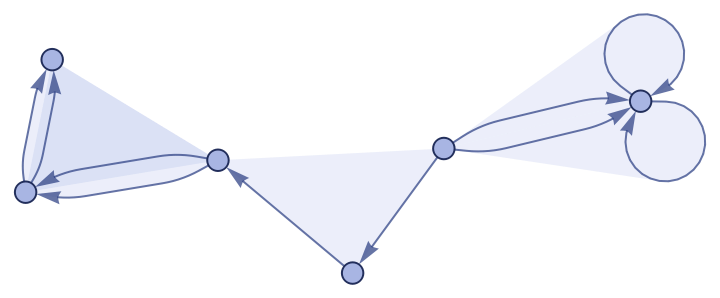Options (17)

GraphHighlight (1)

Highlight some vertices and edges:

 In:=Out=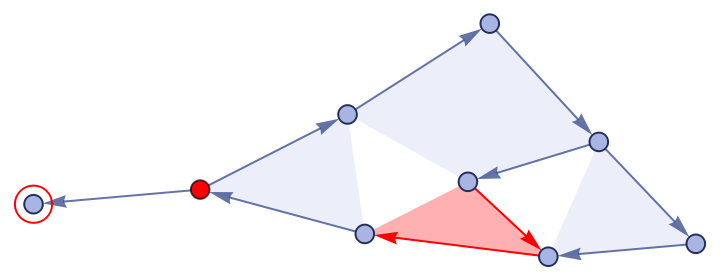GraphHighlightStyle (1)

Change the color of the highlight:

 In:=Out=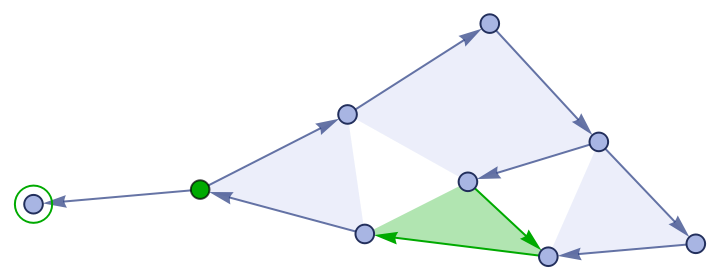HyperedgeRendering (1)

Unroll each hypergraph to a sequence of binary edges:

 In:=Out=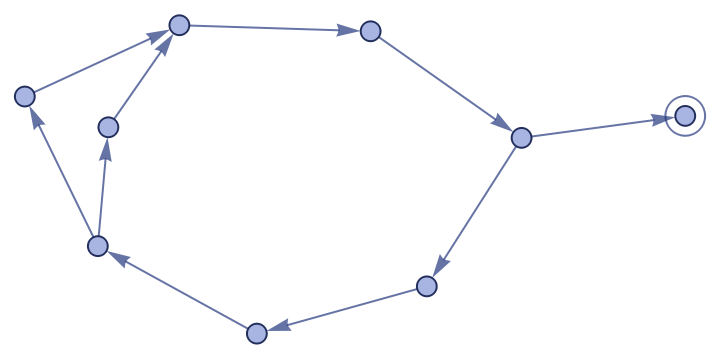VertexCoordinateRules (1)

Specify custom coordinates for the vertices:

 In:=Out=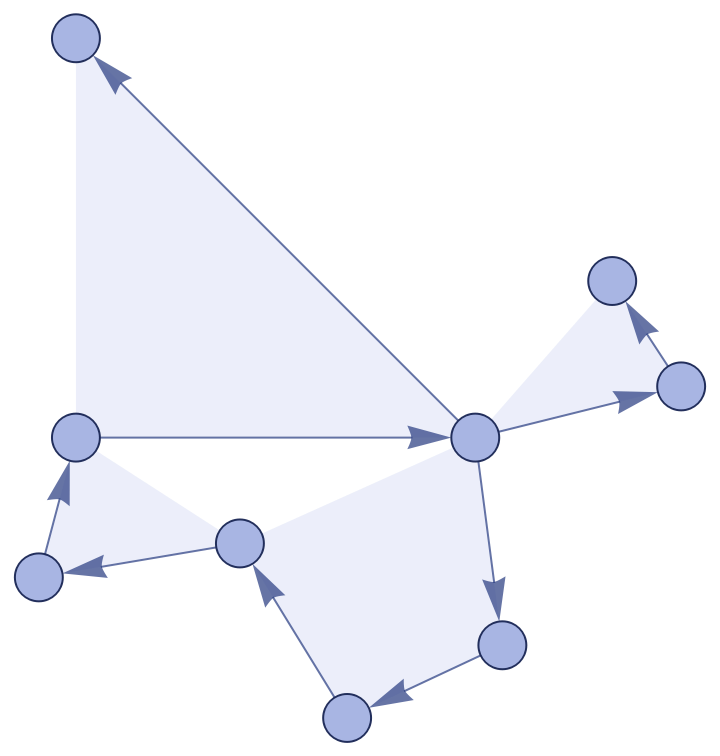VertexLabels (1)

Display the labels for vertices:

 In:=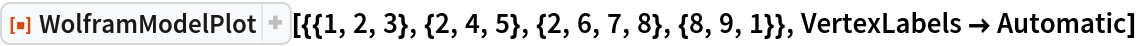Out=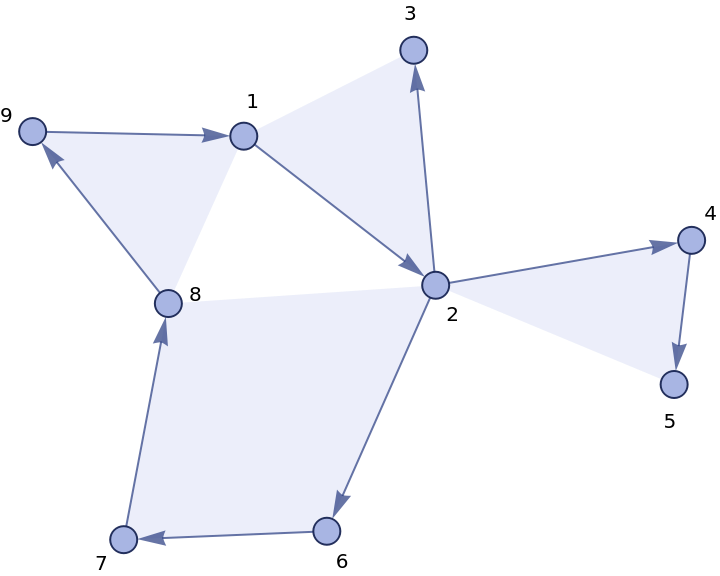VertexSize (1)

Make vertices appear larger:

 In:=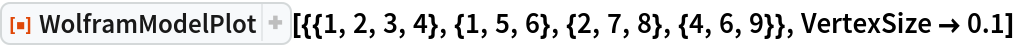Out=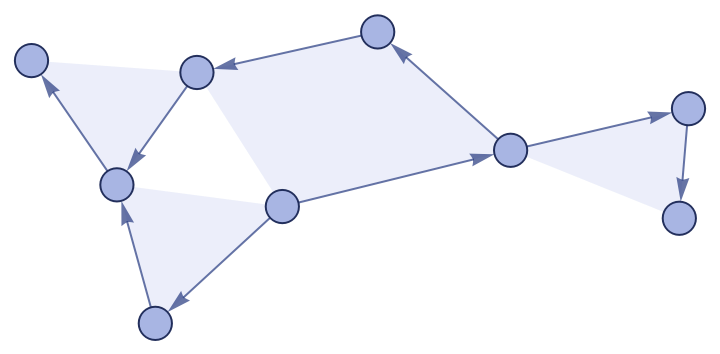In:=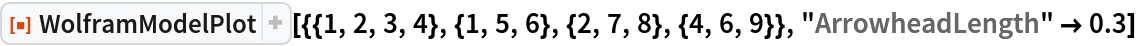Out=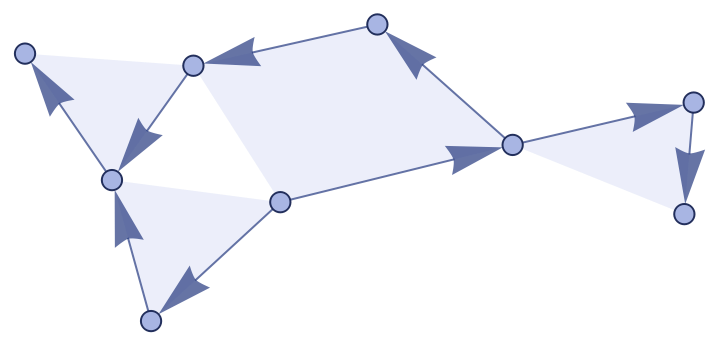MaxImageSize (1)

Reduce the maximum image size, but keep small hypergraphs small:

 In:=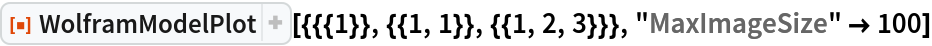Out=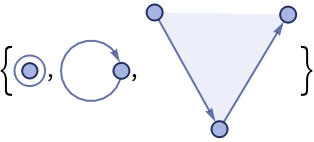PlotStyle (1)

Specify an overall style:

 In:=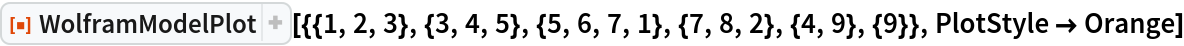Out=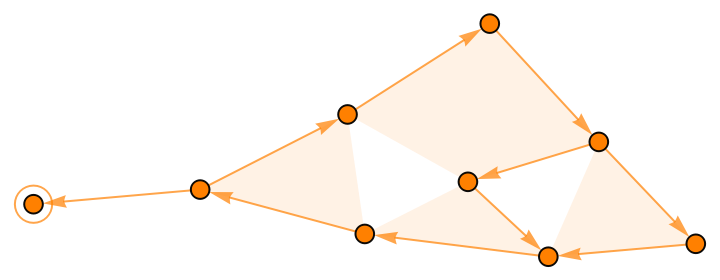VertexStyle (3)

Specify a style for vertices:

 In:=Out=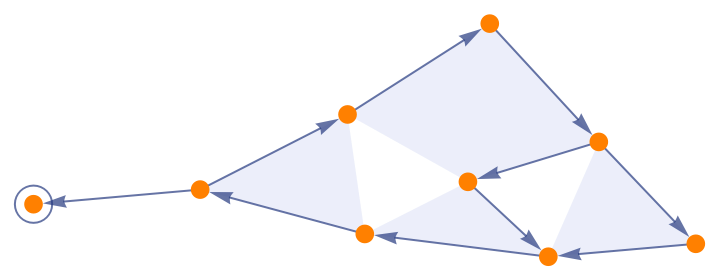Styles for each vertex can be given as a List:

 In:=Out=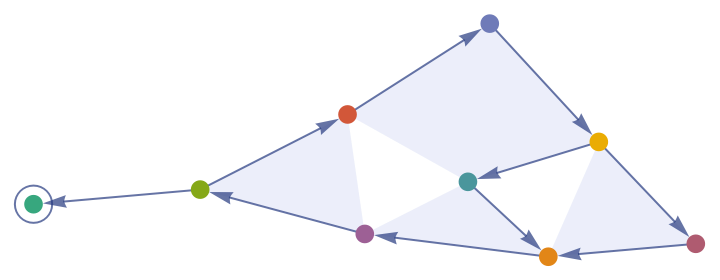Styles for different vertex patterns can be given as an Association:

 In:=Out=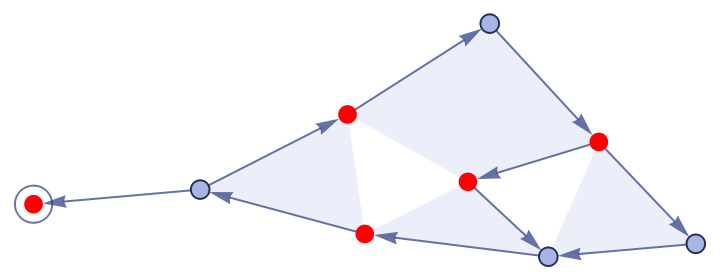EdgeStyle (3)

Specify a style for edges:

 In:=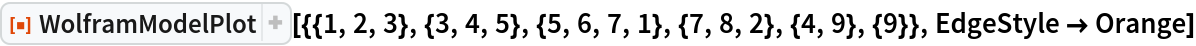Out=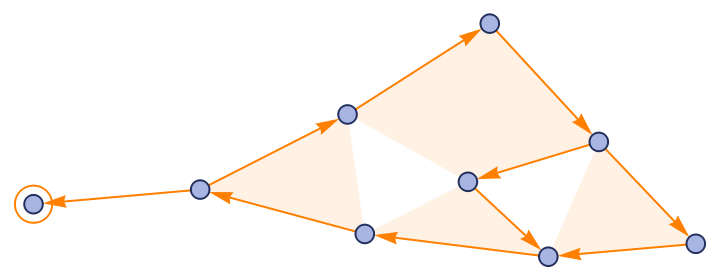Styles for each edge can be given as a List:

 In:=Out=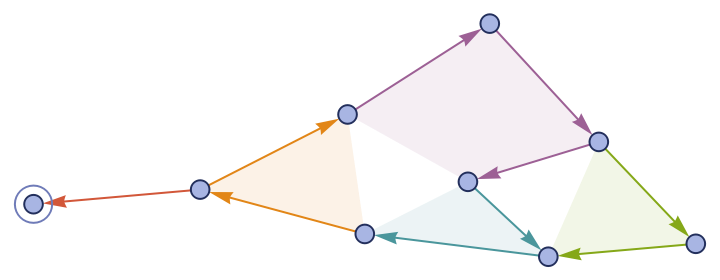Styles for different edge patterns can be given as an Association:

 In:=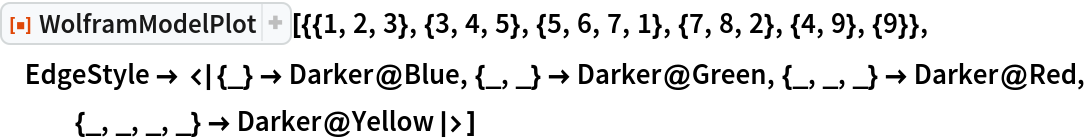Out=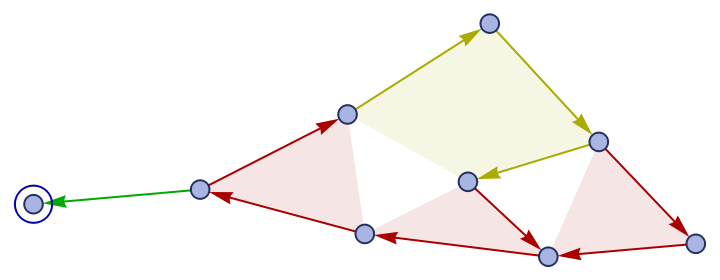EdgePolygonStyle (2)

Specify a style for edge polygons:

 In:=Out=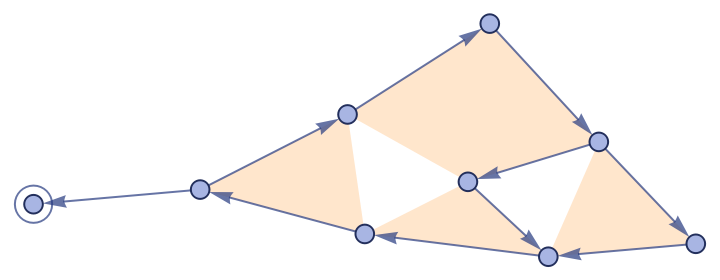This can be specified independently from the style for edge lines:

 In:=Out=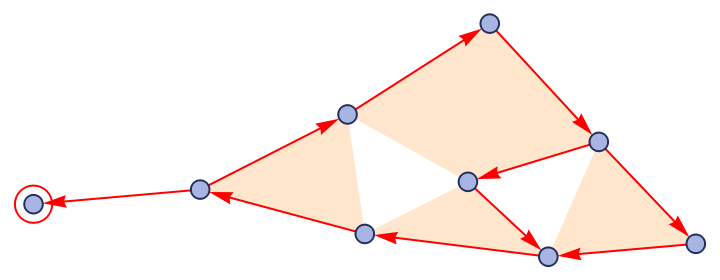Properties and Relations (2)

WolframModelPlot is used in various properties of WolframModel:

 In:=Out=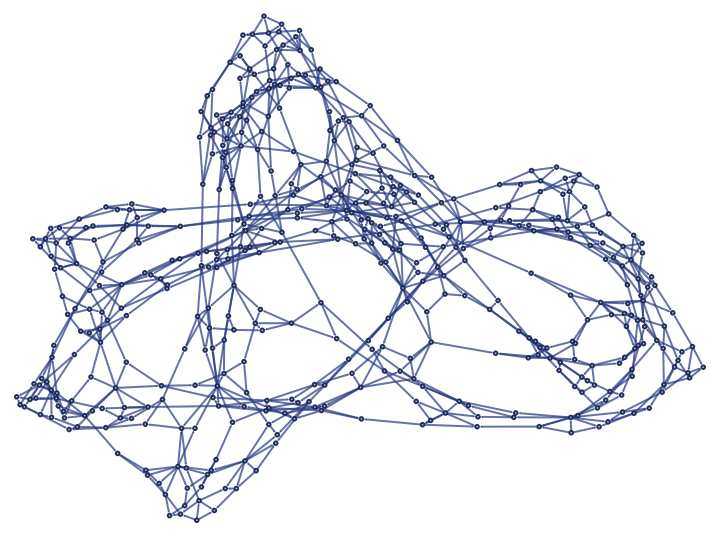It is also used in RulePlot:

 In:=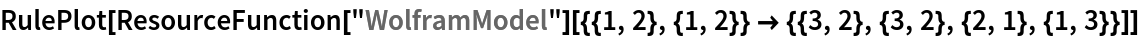Out=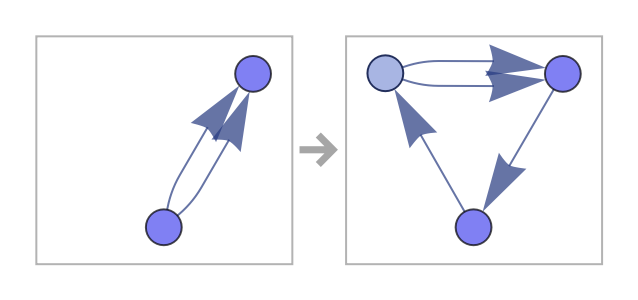Possible Issues (2)

It may be hard to see the boundaries between hyperedges:

 In:=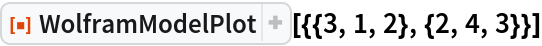Out=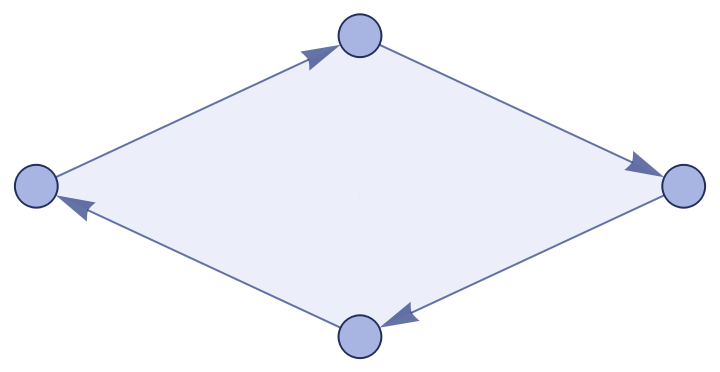Using different colors for each edge might help:

 In:=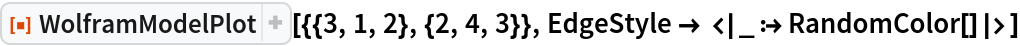Out=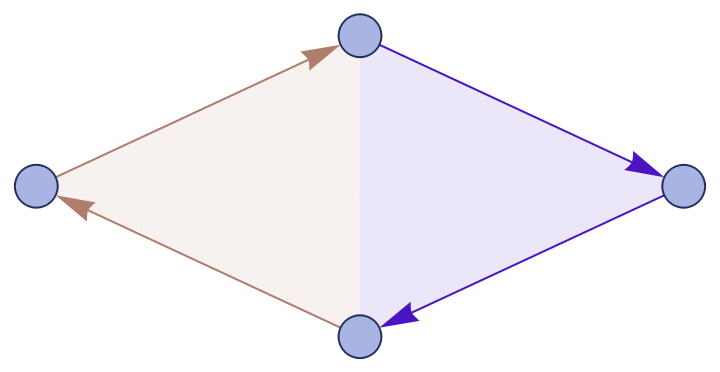Manually specifying coordinates might break the scaling, so vertices and arrowheads may appear too large relative to the distance between vertices:

 In:=Out=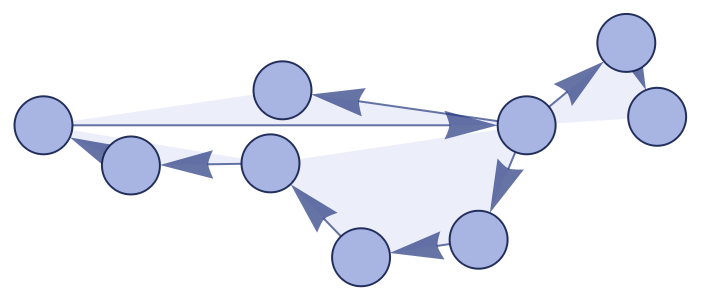Manually reduce the vertex/arrowhead sizes to get a better-looking image:

 In:=Out=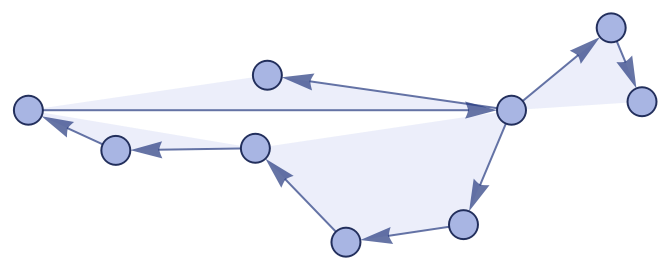Neat Examples (3)

Make polygon art by getting rid of the vertices and arrowheads:

 In:=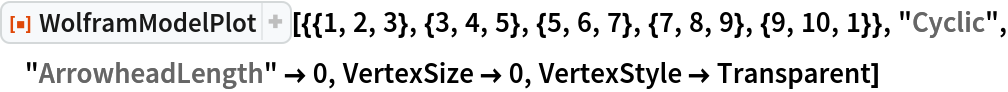Out=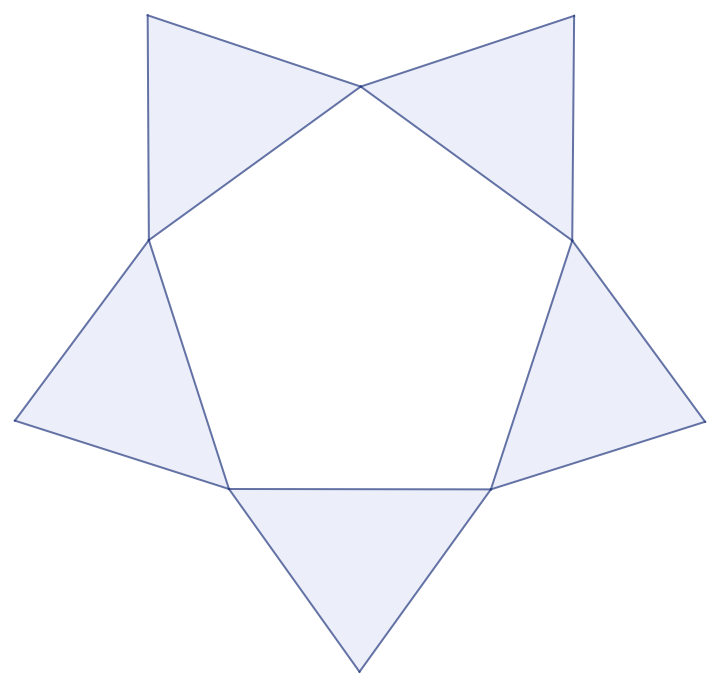Make an unordered hypergraph plot by specifying a custom edge style:

 In:=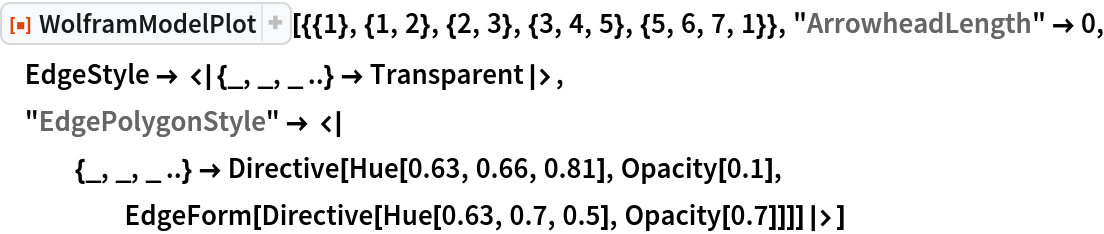Out=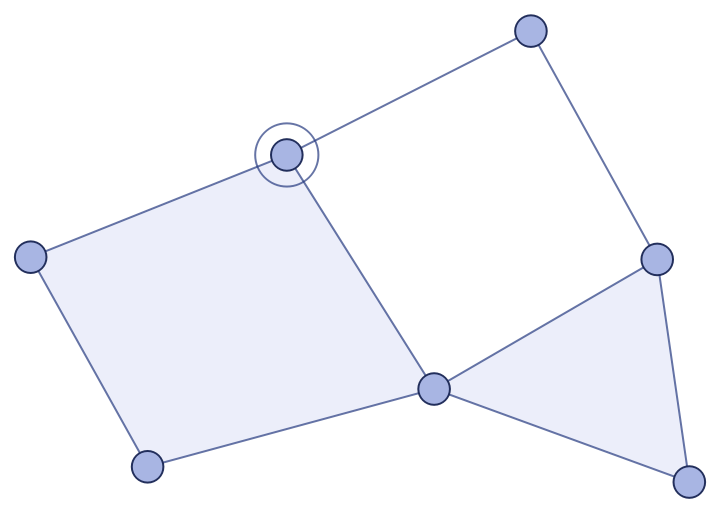Display binary and ternary edges in different colors:

 In:=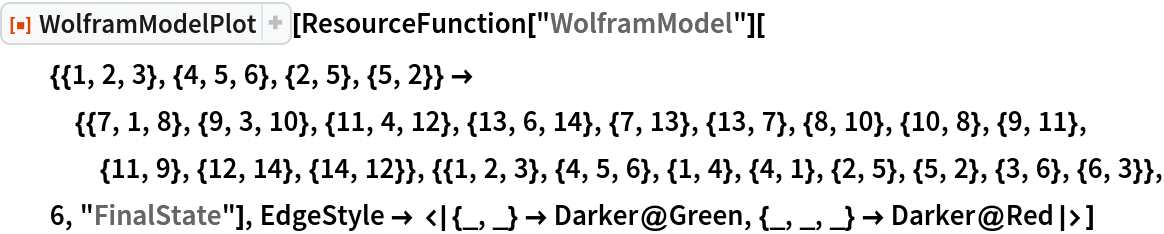Out=